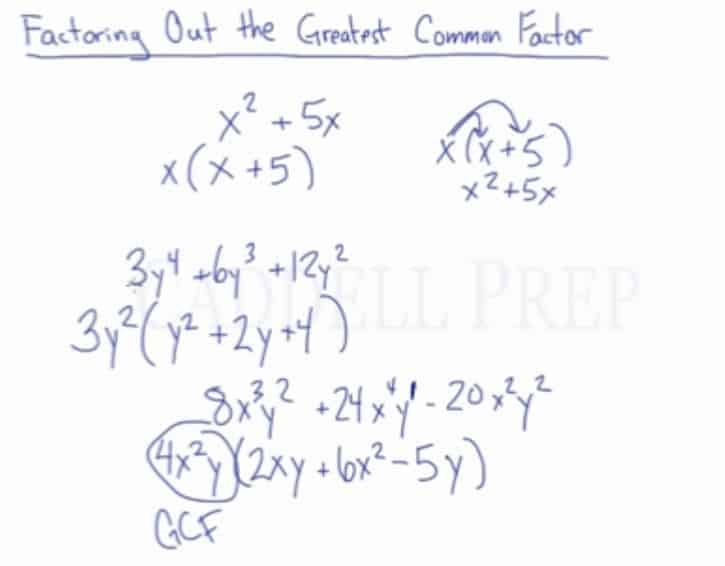Learn how to factor out the greatest common factor (GCF). After you finish this lesson, view all of our Algebra 1 lessons and practice problems.

In this video, we are going to look at how to factor out the greatest common factor.
For example:
To factor:$x^2+5x$
we can factor out a variable, x, by dividing it out of each term, leaving us with$x(x+5)$
To factor:$3y^4+6y^3+12y^2$
the largest number we can pull out from all of the terms is 3, and the largest variable is$y^2$. So if we factor out$3y^2$ then we would be left with$3y^2(y^2+2y+4)$
To factor:$8x^3y^2+24x^4y-20x^2y^2$
the largest number that can be pulled out is 4, the largest variable in terms of x is$x^2$, and the largest variable in terms of y is just y. So if we factor out$4x^2y$then we would be left with$4x^2y(2xy+6x^2-5y)$## Example of Factor Out The Greatest Common Factor (GCF)

### Example 1$15x^3y^2 - 21x^2y^3 + 12x^4y$
The largest number that can be pulled out is$3$, the largest variable in terms of$x$ is$x^2$, and the largest variable in terms of$y$ is just$y$.

So if we factor out$3x^2y$ then we would be left with$3x^2y(5xy-7y^2+4x^2)$

### Example 2$18x^4y^5 + 36x^3y^7$

The largest number that can be pulled out is$18$, the largest variable in terms of$x$ is$x^3$, and the largest variable in terms of$y$ is$y^5$

So if we factor out$18x^3y^5$ then we would be left with$18x^3y^5(x+2y^2)$

## Video-Lesson Transcript

Let’s go over factoring out the greatest common factor.

We have this expression:$x^2 + 5x$

We may need to factor this.

The coefficients are$1$ and$5$.

The greatest common factor of these two is$1$.

But it will not help us. Since when we divided by$1$, we have the same exact expression.

But we can factor out the variable$x$. Both terms can be divided by$x$.

So, we’re taking$x$ out.

When we factor, we divide out whatever the greatest common factor is.

Factoring undoes multiplication.

So when we find the greatest common factor, we divide it out in each term.

So in$x^2 + 5x$,

let’s take$x$ out.

Our answer is$x (x + 5)$.

We could see if we’re actually multiplying this. If we end up with the original expression.

Let’s distribute$x$ into each term in the parenthesis.$x (x + 5)$$x^2 + 5x$

Let’s look at a more complex example$3y^4 + 6y^3 + 12y^2$

First, let’s figure out what the greatest common factor is of the coefficients.

So,$3$,$6$, and$12$.

The biggest number that goes into all of them is$3$.

Here’s a trick:

The greatest common factor of the variable is the term with the lowest exponent.

So in this case,$y^2$ is the greatest common factor of the variable. So let’s take it out.

Now, let’s divide each term by$3y^2$.$3y^4 + 6y^3 + 12y^2$$3y^2 (y^2 + 2y + 4)$

Let’s try an even more complicated example$8x^3y^2 + 24 x^4y - 20x^2y^2$

What’s the greatest common factor of$8$,$24$ and$20$?$4$.$x^2$ and$y$

Now let’s divide$8x^3y^2 + 24 x^4y - 20x^2y^2$ by it’s greatest common factor$4x^2y$$4x^2y (2xy + 6x^2 - 5y)$

To recap, when factoring out the greatest common factor, we have to find the greatest common factor of each of the coefficients. And find what variables are in common with the smallest exponent.

And divide each term by the greatest common factor.

We’ll have a monomial and polynomial.

The monomial being the greatest common factor or GCF.